International
Tables for
Crystallography
Volume F
Crystallography of biological macromolecules
Edited by M. G. Rossman and E. Arnold

International Tables for Crystallography (2006). Vol. F, ch. 18.1, pp. 372-373   | 1 | 2 |

Section 18.1.8. Optimization methods

L. F. Ten Eycka* and K. D. Watenpaughb

aSan Diego Supercomputer Center 0505, University of California at San Diego, 9500 Gilman Drive, La Jolla, CA 92093-0505, USA, and bStructural, Analytical and Medicinal Chemistry, Pharmacia & Upjohn, Inc., Kalamazoo, MI 49001-0119, USA
Correspondence e-mail:  lteneyck@sdsc.edu

18.1.8. Optimization methods

| top | pdf |

Optimization methods for small molecules are straightforward, but macromolecules present special problems due to their sheer size. The large number of parameters vastly increases the volume of the parameter space that must be searched for feasible solutions and also increases the storage requirements for the optimization process. The combination of a large number of parameters and a large number of observations means that the computations at each cycle of the optimization process are expensive.

Optimization methods can be roughly classified according to the order of derivative information used in the algorithm. Methods that use no derivatives find an optimum through a search strategy; examples are Monte Carlo methods and some forms of simulated annealing. First-order methods compute gradients, and hence can always move in a direction that should reduce the objective function. Second-order methods compute curvature, which allows them to predict not only which direction will reduce the objective function, but how that direction will change as the optimization proceeds. The zero-order methods are generally very slow in high-dimensional spaces because the volume that must be searched becomes huge. First-order methods can be fast and compact, but cannot determine whether or not the solution is a true minimum. Second-order methods can detect null subspaces and singularities in the solution, but the computational cost grows as the cube of the number of parameters (or worse), and the storage requirements grow as the square of the number of parameters – undesirable properties where the number of parameters is of the order of 104.

Historically, the most successful optimization methods for macromolecular structures have been first-order methods. This is beginning to change as multi-gigabyte memories are becoming more common on computers and processor speeds are in the gigahertz range. At this time, there are no widely used refinement programs that run effectively on multiprocessor systems, although there are no theoretical barriers to writing such a program.

18.1.8.1. Solving the refinement equations

| top | pdf |

Methods for solving the refinement equations are described in IT C Chapters 8.1to 8.5and in many texts. Prince (1994)provides an excellent starting point. There are two commonly used approaches to finding the set of parameters that minimizes equation (18.1.4.1). The first is to treat each observation separately and rewrite each term of (18.1.4.1)as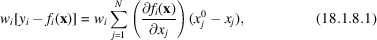where the summation is over the N parameters of the model. This is simply the first-order expansion of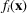and expresses the hypothesis that the calculated values should match the observed values. The system of simultaneous observational equations can be solved for the parameter shifts provided that there are at least as many observations as there are parameters to be determined. When the number of observational equations exceeds the number of parameters, the least-squares solution is that which minimizes (18.1.4.1). This is the method generally used for refining small-molecule crystal structures, and increasingly for macromolecular structures at atomic resolution.

18.1.8.2. Normal equations

| top | pdf |

In matrix form, the observational equations are written as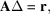where A is the M by N matrix of derivatives, Δ is the parameter shifts and r is the vector of residuals given on the left-hand sides of equation (18.1.8.1). The normal equations are formed by multiplying both sides of the equation by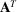. This produces an N by N square system, the solution to which is the desired least-squares solution for the parameter shifts.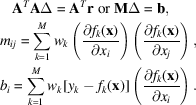Similar equations are obtained by expanding (18.1.4.1)as a second-order Taylor series about the minimum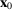and differentiating.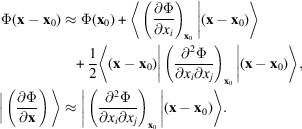The second-order approximation is equivalent to assuming that the matrix of second derivatives does not change and hence can be computed at x instead of at.

18.1.8.3. Choice of optimization method

| top | pdf |

First-order methods are generally the most economical for macromolecular problems. The most general approach is to treat the problem as a non-linear optimization problem from the beginning. This strategy is used by TNT (Tronrud et al., 1987; Tronrud, 1997) and by X-PLOR (Kuriyan et al., 1989), although by very different methods.

TNT uses a preconditioned conjugate gradient procedure (Tronrud, 1992), where the preconditioning function is the second derivatives of the objective function with respect to each parameter. In other words, at each step the parameters are normalized by the curvature with respect to that parameter, and a normal conjugate gradient step is taken. This has the effect that stiff parameters, which have steep derivatives, are scaled down, while soft parameters (such as B factors), which have small derivatives, are scaled up. This greatly increases both the rate and radius of convergence of the method.

X-PLOR (and its intellectual descendent, CNS) (Chapter 18.2and Section 25.2.3) uses a simulated annealing procedure that derives sampling points by molecular dynamics. Simulated annealing is a process by which the objective function is sampled at a new point in parameter space. If the value of the objective function at the new point is less than that at the current point, the new point becomes the current point. If the value of the objective function is greater at the new point than at the current point, the Boltzmann probability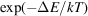of the difference in function values ΔE is compared to a random number. If it is less than the random number, the new point is accepted as the current point; otherwise it is rejected. This process continues until a sufficiently deep minimum is found that the sampling process never leaves that region of parameter space. At this point the temperature' in the Boltzmann factor is reduced, which lowers the probability that the current point will move out of the region. This produces a finer search of the local region. The cooling process is continued until the solution has been restricted to a sufficiently small region. There are many variations of the strategy that affect the rate of convergence and the completeness of sampling. The primary virtue of simulated annealing is that it does not become trapped in shallow local minima. Simulated annealing can be either a zero-order method or a first-order method, depending on the strategy used to generate new sampling points. X-PLOR treats the fit to the diffraction data as an additional energy term, and the gradient of that energy' is treated as a force. This makes it a first-order method.

The first widely available macromolecular refinement program, PROLSQ (Konnert, 1976), uses an approximation to the second-order problem in which the matrix is greatly simplified. The parameters for each atom are treated as a small block on the diagonal of the matrix, and the off-diagonal blocks for pairs of atoms related by geometric constraints are also filled in. The sparse set of linear equations is then solved by an adaptation of the method of conjugate gradients.

The most comprehensive refinement program available for macromolecules is the same as the most comprehensive program available for small molecules – SHELXL98 (Sheldrick, 1993; see also Section 25.2.10). The primary adaptations to macromolecular problems have been the addition of conjugate gradients as an optimization method for cases in which the full matrix will not fit in the available memory and facilities to process the polymeric models required for macromolecules.

18.1.8.4. Singularity in refinement

| top | pdf |

Unless there are more linearly independent observations than there are parameters to fit them, the system of normal equations has no solution. The inverse of the matrix does not exist. Second-order methods fail in these circumstances by doing the matrix equivalent of dividing by zero. However, the objective function is still defined and has a defined gradient at all points. First-order methods will find a point at which the gradient is close to zero, and zero-order methods will still find a minimum value for the objective function. The difficulty is that the points so found are not unique. If one computes the eigenvalues and eigenvectors of the matrix of normal equations, one will find in this case that there are some eigenvalues that are very small or zero. The eigenvectors corresponding to these eigenvalues define sets of directions in which the parameters can be moved without affecting the value of the objective function. This region of the parameter space simply cannot be determined by the available data. The only recourses are to modify the model so that it has fewer parameters, add additional restraints to the problem, or collect more data. The real hazard with this situation is that the commonly used refinement methods do not detect the problem. Careful use of cross validation and keeping careful count of the parameters are the only remedy.

References

Konnert, J. H. (1976). A restrained-parameter structure-factor least-squares refinement procedure for large asymmetric units. Acta Cryst. A32, 614–617.Google Scholar
Kuriyan, J., Brünger, A. T., Karplus, M. & Hendrickson, W. A. (1989). X-ray refinement of protein structures by simulated annealing: test of the method on myohemerythrin. Acta Cryst. A45, 396–409.Google Scholar
Prince, E. (1994). Mathematical techniques in crystallography and materials science. 2nd ed. Berlin: Springer-Verlag.Google Scholar
Sheldrick, G. M. (1993). SHELXL93. Program for the refinement of crystal structures. University of Göttingen, Germany.Google Scholar
Tronrud, D. E. (1992). Conjugate-direction minimization: an improved method for the refinement of macromolecules. Acta Cryst. 48, 912–916.Google Scholar
Tronrud, D. E. (1997). The TNT refinement package. Methods Enzymol. 277, 306–318.Google Scholar
Tronrud, D. E., Ten Eyck, L. F. & Matthews, B. W. (1987). An efficient general-purpose least-squares refinement program for macromolecular structures. Acta Cryst. A43, 489–501.Google Scholar